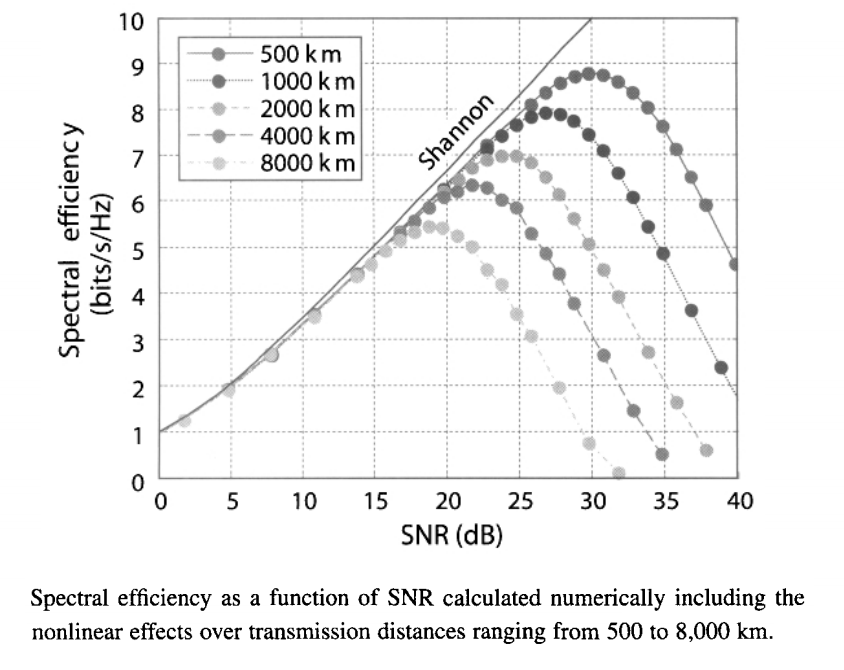# Recent Progress in Phase-Encoded Lightwave Systems

Many transmission experiments performed during the 1980s demonstrated that coherent receivers could function at lower power levels compared with that required for direct detection. With the advent of optical amplifiers, this issue became irrelevant. However, as WDM systems began to transmit more and more channels within the 40-nm bandwidth of the C band, the issue of spectral efficiency led after 2001 to a renewed interest in phase-encoded lightwave systems. This section reviews the recent progress realized in designing such systems.

#### 1. Systems with the DBPSK Format

The DBPSK format was the first one employed for demonstrating high-capacity WDM systems. The reason is related to the adoption of the delay-demodulation scheme of the demodulation schemes tutorial based on an optical interferometer; with its use, receiver design is similar to that employed for IM/DD receivers. Moreover, optical transmitters for generating a DBPSK signal require only one additional phase modulator. However, the DBPSK format allows one to send only one bit per symbol, resulting in a symbol rate equal to the bit rate. Since any gain in spectral efficiency is relatively modest, why should one use the DBPSK format in place of the standard OOK format? It turns out that the nonlinear XPM effects are reduced considerably with the use of DBPSK. As a result, this format improves the system performance considerably for dense WQDM systems designed to provide a spectral efficiency ηs > 0.4 b/s/Hz.

This advantage was realized in 2002 when 64 WDM channels, each operating at 42.7 Gb/s, were transmitted over 4000 km using the RZ-DBPSK format. The use of 100-GHz channel spacing resulted in a 53-nm bandwidth of the DWDM signal with ηs = 0.4 b/s/Hz. By 2003, several experiments reported major advances by employing the DBPSK format. In one experiment, the spectral efficiency of ηs = 0.8 b/s/Hz was realized by transmitting 64 WDM channels, each operating at 42.7 Gb/s with a channel spacing of only 50 GHz, over 3200 km of fiber, resulting in a system capacity of 6.4 Tb/s. In another, the objective was to demonstrate the transmission of 40 channels (each at 40 Gb/s) over a trans-Pacific distance of 10,000 km. These two experiments used a format known as CSRZ-DBPSK, where CSRZ stands for carrier-suppressed RZ, in which two neighboring RZ pulses have a relative phase difference of π, in addition to the phase shift required by the bit pattern. Another experiment transmitted 185 WDM channels, each operating at 10.7 Gb/s, over 830 km using the RZ-DBPSK format. Still, another transmitted 373 WDM channels, each operating at 10.7 Gb/s, over 11,000 km using the same format. Channels were spaced apart by 25 GHz (ηs = 0.4 b/s/Hz) and occupied an 80-nm bandwidth in the 1.55-μm spectral region. In a later experiment, spectral efficiency could be increased to 0.65 b/s/Hz, but the number of channels was limited to 301.

Spectral efficiency in the preceding experiments was limited to 0.8 b/s/Hz. However, a 2002 experiment managed to realize a spectral efficiency of 1.6 b/s/Hz by combining 40 DBPSK channels (each at 40 Gb/s) with optical code-division multiplexing, resulting in a system capacity of 6.4 Tb/s. The bit rate of 160 Gb/s per channel was realized in a 2003 experiment in which six such channels were transmitted over 2000 km, with spectral efficiency of 0.53 b/s/Hz, using the RZ-DBPSK format. Of course, the 160-Gb/s signal containing 3.5-ps pulses has to be generated through an OTDM scheme. This experiment showed that the DBPSK format could be used even at a bit rate of 170.6 Gb/s that is required for 160-Gb/s systems when a forward-error correction scheme is implemented.

The advantages of the DBPSK format were quantified in a 2003 WDM experiment that transmitted 100 channels over transoceanic distances with spectral efficiency of 0.22 b/s/Hz. The experiment compared the DBPSK and OOK formats using both the RZ and NRZ bit streams at a bit rate of 10.7 Gb/s. Figure (a) below shows the Q factors as a function of optical SNR (measured in a 0.1-nm bandwidth) for the 4 formats with noise added by an amplifier (before transmission). Figure (b) shows the degradation in Q factors as a function of transmission distance with the same launched average power in all cases (Q values were averaged over three widely separated channels). The RZ-DPSK format is superior initially to all other formats, and this advantage is retained up to a distance of about 6300 km, after which RZ-OOK has a slightly higher Q factor. A faster reduction in Q for the RZ-DBPSK format indicates that the nonlinear effects were more serious in the latter case. However, this situation changes for dense WDM systems with a spectral efficiency ηs > 0.4 b/s/Hz. The reason appears to be that the XPM-induced degradation under such conditions becomes more severe for systems employing the OOK format.

#### 2. Systems with the DQPSK Format

An obvious advantage of the QPSk format is that it transmits 2 bits/symbol, resulting in a symbol rate that is only 50% of the actual bit rate and a doubling of the spectral efficiency ηs. As a result, QPSk format allows, in principle, ηs > 1 b/s/Hz. This feature led to a large number of experiments dealing with the DQPSK format. In a 2002 experiment, 9 WDM channels were transmitted over 1000 km using a 25-GHz channel spacing with the RZ-DQPSK format. The symbol rate of 12.5 Gbaud corresponded to a bit rate of 25 Gb/s, resulting in ηs = 1 b/s/Hz. (if we ignore the overhead imposed by forward-error correction).

By 2003, an experiment used the DQPSK format to realize a spectral efficiency of 1.6 b/s/Hz. It was able to transmit 8 channels at 40 Gb/s over 200 km with a channel spacing of 25 GHz. The symbol rate was only 10 Gbaud in this experiment because it also employed the PDM or dual-polarization technique in which two orthogonally polarized bit streams are generated at half the original bit rate. The combination of DQPSK and PDM reduces the symbol rate to one-quarter of the actual bit rate and thus enhances the spectral efficiency by a factor of 4. As a result, a 40-Gb/s signal can be transmitted using components developed for 10-Gb/s channels.

The fiber-link length was limited to only 200 km in the preceding 2003 experiment. This limitation is mainly due to the use of PDM. In a 2004 experiment, 64 WDM channels at a symbol rate of 12.5 Gbaud could be transmitted over 6500 km using the RZ-DQPSK format (without PDM) with spectral efficiency of 1 b/s/Hz. The distance could be increased to 10,200 km in a 2005 experiment in which mid-span OPC was employed to compensate fiber dispersion and to reduce simultaneously the impact of nonlinear phase noise. However, the total capacity of this system was only 0.88 Tb/s. A much higher capacity of 5.94 Tb/s was realized in another 2005 experiment that used DQPSK with PDM, but the link length was then limited to 324 km. The link length could be extended to 1700 km (with 1-dB margin) in a 2006 experiment that transmitted 40 channels at a bit rate of 85.6 Gb/s on a 50-GHz frequency grid. This experiment also showed that the QA factors of the received signal were lower by 2.2 dB when the PDM technique was employed, and they decreased linearly with distance whether PDM was used or not.

In recent years, the total system capacity of DQPSK systems has exceeded 10 Tb/s. A capacity of 14 Tb/s was realized in a 2006 experiment that transmitted PDM 140 channels (each operating at 111 Gb/s) within a 59-nm-wide wavelength window extending from 1561 to 1620 nm. This experiment demonstrated a spectral efficiency of 2.0 b/s/Hz over a limited link length of 160 km. The system capacity was increased to 20.4 Tb/s within a year by expanding the wavelength range from 1536 to 1620nm. The link length was 240 km with the same spectral efficiency. A system capacity of 25.6 Tb/s was realized in a 2007 experiment that transmitted 160 WDM channels over 240 km on a 50 GHz grid with spectral efficiency of 3.2 b/s/Hz.

#### 3. QAM and Related Formats

The BPSK and QPSk formats discussed so far in this tutorial encode the data in the phase of the optical carrier but they leave its amplitude unchanged fro one symbol to next. In contrast, the QAM format employs both the amplitude and phase for data encoding. Moreover, the number of symbols (M = 2m) employed can vary from 2 to more than 128 depending on the value of the integer m. An example of the 16-QAM format is shown in the following figure with 16 symbols (m = 4). In this notation, QPSK corresponds to 4-QAM.A major difference from the cases of DBPSK and DQPSk formats is that the use of the QAM format requires coherent detection of the transmitted signal at the receiver end. A phase-locked loop is often used for this purpose, but phase synchronization between the local oscillator and the transmitter laser is a challenging task because of intrinsic phase noise of the two lasers. This task becomes even more challenging in the presence of nonlinear phase noise. Nevertheless, such phase-locking techniques were developed after 2003. In a different approach, a phase-diversity homodyne scheme was employed without a phase-locked loop and the carrier phase was estimated with (DSP) on the homodyne signal. The following figure shows the design of such a digital coherent receiver. An analog-to-digital converter is used to convert the filtered analog signal into a digital format suitable for DSP. Because of the use of DSP, such an approach can also compensate for distortions of the optical signal induced inside the fiber link through mechanisms such as chromatic dispersion.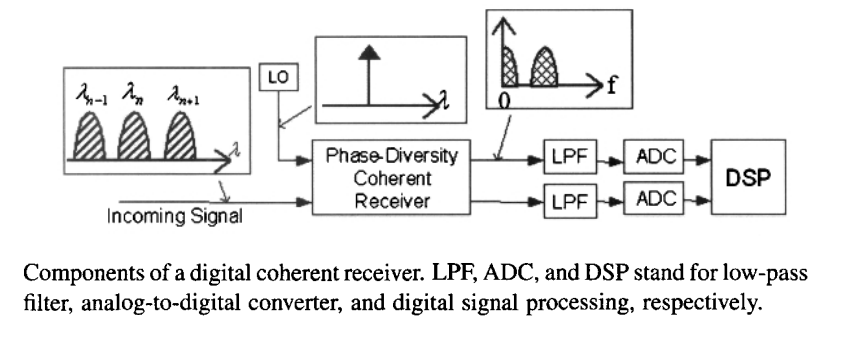In a 2005 experiment, coherent demodulation of a 40-Gb/s bit stream, encoded using the QPSK (or 4-QAM) format with polarization multiplexing and transmitted over 200 km, had been realized. Because the symbol rate was 10 Gbaud, three WDM channels could be separated by only 16 GHz. The experiment employed a phase-diversity coherent receiver with digital signal processing (DSP). By 2008, 164 channels at 111 Gb/s were transmitted over 2550 km at a symbol rate of 27.75 Gbaud by combining the QPSK format with polarization multiplexing. The channel spacing of 50 GHz required the use of both the C and L bands, but the experiment realized a record capacity-distance product of 41,800 (Tb/s)-km, while employing coherent detection. The following below shows the design and the photograph of the digital coherent receiver used in this experiment; the inset shows the compact size that was possible because of the use of DSP within the receiver. By 2010, the dual-polarization QPSK format was in use for commercial systems.Spectral efficiency of such systems can be improved further by transmitting more than 2 bit/symbol. In a 2006 experiment, a 128-QAM signal at 20 Mbaud was transmitted over 525 km and then detected using heterodyne detection with a frequency-stabilized fiber laser and an offset-locking technique. This experiment did not employ the WDM technique, but it showed that up to 7 bits per symbol could be encoded successfully with the QAM format. In a later experiment, the symbol rate was increased to 1 Gbaud, and a 64-QAM signal was transmitted over 150 km of fiber. This experiment employed heterodyne detection with a frequency-stabilized fiber laser and used an optical phase-lock loop. By 2008, this scheme was extended to transmit three WDM channels (each operating at 12 Gb/s) over 160 km with only 1.4-GHz channel spacing, resulting in a spectral efficiency of 8.6 b/s/Hz. In another experiment, a single 40-Gb/s channel was transmitted over 100-km of standard fiber with the 16-QAM format and demodulated using a digital coherent receiver.

The state of the art has improved considerably for QAM-based WDM systems in recent years. In a 2008 experiment, 10-channels (each operating at 112 Gb/s) with 25-GHz spacing were transmitted over 315 km using the 16-QAM format with PDM, resulting in a symbol rate of 14 Gbaud and a spectral efficiency of 4.5 b/s/Hz. By 2009, the fiber length was increased to 630 km, while the channel spacing could be reduced to 16.7 GHz so that the spectral efficiency was 6.7 b/s/Hz. If the FEC overhead is taken into account and the actual bit rate of 104 Gb/s is used, the spectral efficiency becomes 6.2 b/s/Hz, still a very respectable number. A high-capacity system was demonstrated in 2008 by transporting 161 channels, each operating at a bit rate of 114 Gb/s, over 662 km of fiber. It combined the 8-PSK format with polarization multiplexing, resulting in a symbol rate of 19 Gbaud. All 114 channels could be amplified by a single C-band EDFA because of the 25-GHz channel spacing employed in this experiment. A system capacity of 32 Tb/s was realized in a 2009 experiment that transmitted 320 WDM channels (each at 114 Gb/s) over 580 km of fiber by using the 8-QAM format with PDM. By 2010, system capacity was more than doubled to 69.1 Tb/s by transmitting 432 channels (each at 171 Gb/s) over 240 km with a spectral efficiency of 6.4 b/s/Hz by using the 16-QAM format. Another 2010 experiment realized 64-Tb/s capacity by transmitting 640 channels (each at 107 Gb/s) over 320 km with a spectral efficiency of 8 b/s/Hz in the 32-QAM format.

#### 4. Systems Employing Orthogonal FDM

As discussed in the subcarrier multiplexing (SCM) tutorial, orthogonal FDM, or OFDM, is a subcarrier multiplexing technique that makes use of the FFP algorithm with DSP to transmit multiple bits simultaneously at orthogonal subcarrier frequencies located in the vicinity of the main carrier. This technique is widely used for cellular transmission at microwave frequencies and has recently been adopted for lightwave systems because it has the potential of improving their performance considerably. Its main advantage is that the OFDM symbol rate is a small fraction of the actual bit rate because hundreds of bits are transmitted in parallel using multiple subcarriers separated by 1/Ts. Moreover, no dispersion compensation is typically required because dispersion-induced distortions can be removed at the receiver through DSP of the electrical signal in the frequency domain.

The coherent optical OFDM technique was proposed in 2005 and its advantages were studied soon after. An experimental demonstration of this technique at a bit rate of 8 Gb/s employed 128 subcarriers with the QPSK format. Such an optical OFDM signal was transmitted over 1000 km of standard telecommunication fiber (using a recirculating fiber loop) without any dispersion compensation. This experiment employed two narrowband lasers (one at the transmitter and the other at the receiver) with line widths of around 20 kHz. Such narrowband lasers are needed because of a relatively low symbol rate of subcarriers and the coherent detection used for them.

A transmission distance of 4160 km at a bit rate of 25.8 Gb/s was realized in a 2007 OFDM experiment in which 256 subcarriers were used. This experiment implemented a phase-noise compensation scheme by inserting a radio-frequency (RF) pilot at the transmitter. Since this pilot is distorted by phase noise in exactly the same way as the OFDM signal, it can be used at the receiver to remove phase distortions from the OFDM signal. The following figure shows the effectiveness of this technique by comparing constellation diagrams with and without compensation of the phase noise using an RF pilot. An external-cavity semiconductor laser with a 100-kHz linewidth was used as the local oscillator at the receiver end.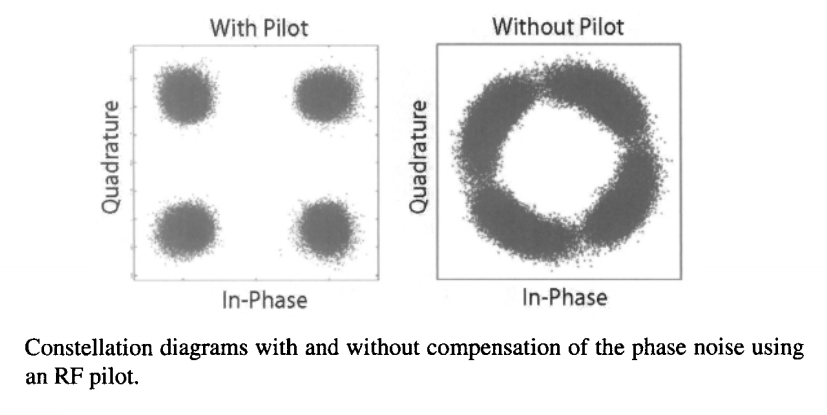Some OFDM systems focus on the bit rate of 100 Gb/s that is required for the 100-GbE standard. The actual bit rate is slightly higher in practice because of the FEC overhead. By 2008, a bit rate of 107 Gb/s was realized for an OFDM system operating with 128 QPSK-encoded subcarriers. The resulting OFDM signal could be transmitted over 1000 km of standard fiber without any dispersion compensation in the optical domain. The 37-GHz bandwidth of the OFDM signal led to a spectral efficiency of close to 3 b/s/Hz. In another experiment, a spectral efficiency of 2 b/s/Hz was realized by transmitting ten 121.9-Gb/s WDM channels over 1000 km of standard fiber through OFDM with 50-GHz channel spacing. This experiment employed the 8-QAM format with PDM such that each 121.9 Gb/s channel occupied a bandwidth of only 22.8 GHz.

The spectral efficiency was increased to 4 b/s/Hz in a 2009 experiment that reduced the channel spacing to 25 GHz. In this experiment, seven 132.2-Gb/s channels were transmitted over 1300 km of standard fiber using the 8-QAM format with PDM. The symbol length was 14.4 ns in the case of 128 subcarriers but it increased to 104 ns for 1024 subcarriers. The figure below shows the observed BER for the central channel after 1000 km of transmission as a function of the average input power/channel for three symbol lengths corresponding to the use of 128, 256, and 1024 subcarriers. The linear phase distortions were compensated in all cases using the RF-pilot technique, resulting in the same system performance at low input powers. However, as the channel power increased to beyond 0.2 mW, nonlinear phase distortions led to a considerable increase in BER in the case of 104-ns symbol length (1024 subcarriers). The BER was the lowest in the case of 128 subcarriers. One reason for this dependence on the symbol length is that the RF pilot is also affected by the SPM and XPM phenomena as the channel power is increased. Such nonlinear distortions of the RF pilot may reduce the effectiveness of the phase-noise compensation scheme.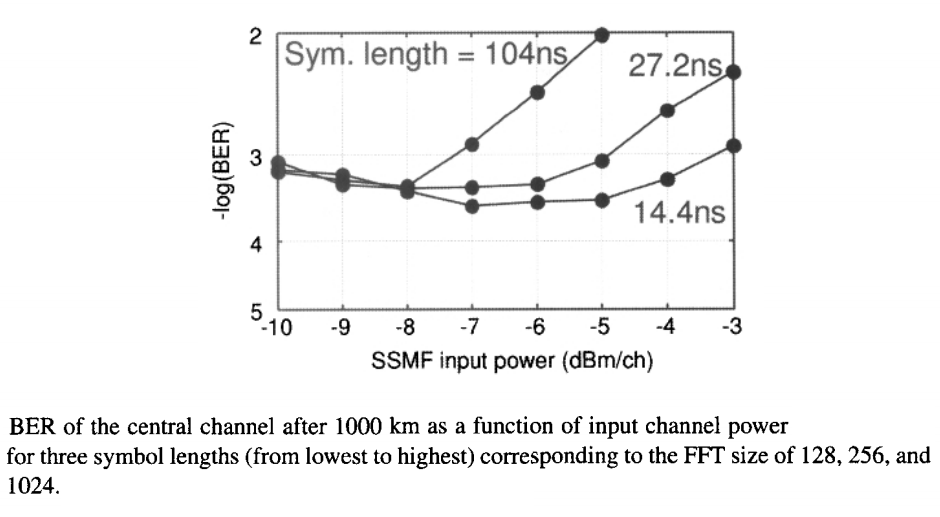The research on optical OFDM systems is progressing rapidly in several directions. In one case, the objective is to enhance the spectral efficiency of WDM systems. In a 2009 experiment, a spectral efficiency of 7 b/s/Hz was realized by transmitting 8 channels, each operating at 65.1 Gb/s, over 630 km of fiber by employing the 32-QAM format with PDM. Channel spacing was only 8 GHz in this WDM experiment. In another case, the objective was to increase the system capacity. In a 2009 experiment, OFDM was used to transmit 135 channels, each operating at 111 Gb/s, over 6248 km, resulting in a record capacity-distance product of 84,300 (Tb/s)-km. This experimetn employed the QPSK format and PDM together with distributed Raman amplification for the WDM signal extending from 1563 to 1620 nm.

Motivated by the future 1 Tb/s Ethernet systems, still another direction for lightwave systems is to transmit a single channel at a bit rate of 1 Tb/s or more. Both the OTDM and OFDM techniques can be employed for this purpose. In the case of OFDM, such an objective requires the use of a large number of subcarriers so that the symbol rate per subcarrier is reasonable. In one 2009 experiment, 4104 spectrally overlapping subcarriers were employed to transmit a 1-Tb/s single channel over 600 km of standard fiber. The entire OFDM signal occupied a bandwidth of 320.6 GHz, resulting in a spectral efficiency of 3.3 b/s/Hz. By 2010, OFDM systems were capable of operating at bit rates of up to 10.8 Tb/s.

#### 5. Ultimate Channel Capacity

With the advent of wavelength-division multiplexing (WDM) technology, lightwave systems with a capacity of more than 1 Tb/s have become available commercially. Moreover, a system capacity of 69.1 Tb/s has been demonstrated in a 2010 laboratory experiment. However, any communication channel has a finite bandwidth, and optical fibers are no exception. One may thus ask what limits the ultimate capacity of a fiber-optic communication system.

The performance of any communication system is ultimately limited by the noise in the received signal. This limitation can be stated more formally by using the concept of channel capacity introduced by Shannon in the context of information theory. It turns out that a maximum possible bit rate exists for error-free transmission of a binary digital signal in the presence of Gaussian noise. This rate is called the channel capacity. More specifically, the capacity (in bits/s) of a noisy communication channel of bandwidth W is given by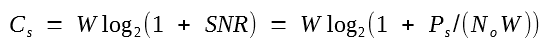where N0 is the spectral density of noise and Ps is the average signal power related to the pulse energy in a symbol as Es = Ps/W.

This equation is valid for a linear channel with additive noise. It shows that the channel capacity (or the bit rate) can exceed channel bandwidth if the noise level is low enough to maintain a high signal-to-noise ratio (SNR). It is common to define the spectral efficiency of a WDM channel as ηs = Cs/W that is a measure of bits transmitted per second per unit bandwidth and is measured in units of (b/s)/Hz. For an SNR > 30 dB, ηs exceeds 10 (b/s)/Hz according to the equation above.

It may appear surprising that the equation above does not depend on the modulation format. We have seen in the advanced modulation formats tutorial that the number of bits that can be transmitted during each symbol is limited by the number of symbols in the constellation diagram (or the alphabet size). In fact, spectral efficiency is limited to log2M for an alphabet size of M. The reason why the equation above is independent of M is that this equation is derived in the limit M → ∞ such that symbols occupy the entire two-dimensional space in the constellation diagram with a Gaussian density distribution. Thus it is important to keep in mind that the following discussion of channel capacity represents an upper limit of what can be realized in practice.

Even with this caveat, the equation above does not always apply to fiber-optic communication systems because of the nonlinear effects occurring inside optical fibers. It can nonetheless be used to provide an upper limit on the system capacity. The total bandwidth of modern lightwave systems is limited by the bandwidth of optical amplifiers and is below 10 THz (80 nm) even when both the C and L bands are used simultaneously. With the advent of new kinds of fibers and amplification schemes, one may expect that eventually this bandwidth my approach 50 THz if we use the entire low-loss region extending from 1.25 to 1.65 μm. If we divide this bandwidth into 1000 WDM channels, each 50 GHz wide, and assume that the SNR exceeds 100 for each channel, the maximum system capacity predicted by the equation above is close to 350 Tb/s, assuming that the optical fiber acts as a linear channel. The highest capacity realized for WDM systems was 69.1 Tb/s in a 2010 experiment. The preceding estimate shows that there is considerable room for improvement. The most limiting factor in practice is the spectral efficiency set by the alphabet size M. The use of a larger M should improve the capacity of future WDM systems.

The impact of nonlinear effects on the channel capacity of lightwave systems has attracted attention in recent years. A systematic approach was developed in a 2010 review on this topic. The following figure shows how the nonlinear effects reduce the ultimate spectral efficiency from its value predicted by the equation above when high signal powers are launched to ensure a high SNR at the receiver in spite of the buildup of amplifier noise along the fiber link. As one may expect, the spectral efficiency depends on the transmission distance, and it becomes worse as this distance increases. However, the most noteworthy feature of the figure below is that, for any transmission distance, a maximum value occurs at an optimum value of SNR that changes with distance. For example, the maximum spectral efficiency for a 1000-km-long link is limited to below 8 (b/s)/Hz, irrespective of the alphabet size of the modulation format employed. This is in sharp contrast to the prediction of the equation above and reflects a fundamental limitation imposed by the nonlinear effects.